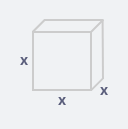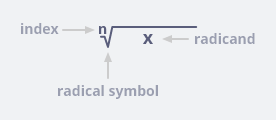Got Feedback?

Found a bug? Have a suggestion? Fill the form below and we'll take a look!

X

Radical calculator is the unique tool for solving the any radical value and expression. This calculator follows the basic rules which help in the simplification of radical (the product and the power laws of the exponents besides nth root of a quotient).

Radical calculator have an ability to solve any radical equation. In short it is the best tool for solving simplifying any radical number and expression.

Radical calculator mechanism is very simple and fast it cater the all aspect of solution and provide quick and accurate results. It facilitate the economy, especially banking sectors, financial institution for estimating the rate of interest and depreciation.

$$y \cdot \sqrt[n]{x} = \; ?$$

### Results:

 Answer: $$= \sqrt{62208}$$ $$= \; \sqrt{7776 \cdot 8}$$ $$= \; 6 \cdot \sqrt{8}$$ $$= \; 6 \, \sqrt{8}$$

### Solution:

 Solution by prime factorization: = $$\sqrt{62208}$$ $$= \sqrt{2^{8} \cdot 3^{5}}$$ $$= 2^{1} \cdot 3^{1} \cdot \sqrt{2^{3}}$$ $$= 2 \cdot 3 \cdot \sqrt{8}$$ $$= \; 6 \, \sqrt{8}$$“Radical is an expression of root”

A radical is an expression having the root or radical symbol. A radical expression can also be re-written as an expression with fraction/ rational exponent.

The number outside the radical symbol (nth root) is called index, the number/expression inside the radical symbol is called the radicand. The basic rules which help in the simplification of radical include the product and the power laws of the exponents besides nth root of quotient.

A radical sign, which looks like a check mark with a roof is used to indicates what number multiplied by itself a certain number of times will give the final answer.

Let, a square with side “x”

Area of Square = A$$A = x^2$$

$$\sqrt{A} = x$$

Volume of Square = V$$v = x^3$$

Exponent 3 becomes the index

$$\sqrt{v} = x$$

For proper understanding, let take a whole picture.Remember radical is an expression for a root.

An equation involving an algebraic expression under the radical sign is called radical equation.

## What is the algebraic expression?

It is the combination of constant and variable connected by mathematical operations.

## Simplifying radicals (factoring the numbers)

Considering few examples

1- $$\sqrt{48}$$

 2 48 2 24 2 12 2 6 3 3 1

= $$\sqrt{2 . 2 . 2 . 2 . 3}$$

= $$2 . 2\sqrt{3}$$

= $$4\sqrt{3}$$

2- $$\sqrt{72}$$

 2 72 2 36 2 18 3 9 3 3 1

= $$\sqrt{2 . 2 . 2 . 3 . 3}$$

= $$2 . 3\sqrt{2}$$

= $$6\sqrt{2}$$

3- $$\sqrt{96}$$

 2 96 2 48 2 24 2 12 2 6 3 3 1

= $$\sqrt{2 . 2 . 2 . 2 . 2 . 3}$$

= $$2\sqrt{2 . 2 . 3}$$

= $$2\sqrt{12}$$

1- $$\sqrt{50}$$

= $$\sqrt{25 ✕ 2} => 5\sqrt{2}$$

2- $$\sqrt{300}$$

= $$\sqrt{3 ✕ 100} => 10\sqrt{3}$$

3- $$\sqrt{3675}$$

= $$\sqrt{3 ✕ 5^2 ✕ 7^2} => 5 . 7 . \sqrt{3} => 35\sqrt{3}$$

4- $$\dfrac{\sqrt{3675}}{\sqrt{300}}$$

= $$\dfrac{35\sqrt{3}}{10\sqrt{3}} => \dfrac{35}{10} => \dfrac{7}{2}$$

5- $$\sqrt{72}$$

= $$\sqrt{2^2 ✕ 2 ✕ 3^2} => 2 . 3 . \sqrt{2} => 6\sqrt{2}$$

Note:- some roots can be represented by integers, but most roots cannot be represented by integers.
For example, $$\sqrt{49} = 7$$ and $$\sqrt{50} = 7.071$$
“Integer is a number which is not a fraction (a whole number)”

## Simplifying expression with rational exponents

Remember, we can simplify them, just like normal fraction.

Let’s try to understand by few examples:

1- $$(n^4)^{\frac{3}{2}} => n^{\frac{12}{2}} => n^6$$

2- $$(27P^6)^{\frac{5}{3}} => 27^{\frac{5}{3}} (P^6)^{\frac{5}{3}} => (3^3)^{\frac{5}{3}} (P^6)^{\frac{5}{3}} => 3^5 P^10 => 243P^10$$

3- $$(P^{\frac{3}{2}})^{-2} => P^{\frac{-6}{2}} => P^{-3} => \frac{1}{P^3}$$

4- $$3 b^{\frac{1}{2}} b^{\frac{4}{3}} => 3 b^{\frac{1}{2} + \frac{4}{3}} => 3 b^{\frac{3 + 8}{6}} => 3 b^{\frac{11}{6}}$$

## Simplifying the expression by applying properties of exponent

1- $$(\dfrac{2 x^3 y^2}{-x^2 y^5})^{-2}$$

Step# 1:

Applying the property of exponent, i-e:

$$(\frac{a}{b})^{-n} = (\frac{b}{a})^{n}$$

Value has negative sign. For this we have to flip the inside value,

= $$(\dfrac{-x^2 y^5}{2 x^3 y^2})^{2}$$

Step# 2:

Apply the product rule, i-e:

$$(ab)^n = a^n b^n$$

Note: eliminate the negative sign as shared exponent is +ve

= $$\dfrac{(x^2)^2 (y^5)^2}{(2)^2 (x^3)^2 (y^2)^2}$$

Step# 3:

Apply power to power rule

= $$\dfrac{x^4 y^{10}}{4 x^6 y^4}$$

Step# 4:

Simplifying the expression by applying exponent property

= $$\dfrac{y^{10-4}}{4 x^{6-4}}$$

= $$\dfrac{y^6}{4 x^2}$$

= $$\dfrac{1}{4} \dfrac{y^6}{x^2}$$

1- $$\dfrac{4\sqrt{3}}{2 + \sqrt{5}}$$

= $$\dfrac{4\sqrt{3}}{2 + \sqrt{5}} ✕ \dfrac{2 – \sqrt{5}}{2 – \sqrt{5}}$$

= $$\dfrac{8\sqrt{3} – 4\sqrt{15}}{4 – 5}$$

= $$\dfrac{8\sqrt{3} – 4\sqrt{15}}{- 1}$$

Taking 4 common

= $$\dfrac{4(2\sqrt{3} – \sqrt{15})}{- 1}$$

= $$-4(2\sqrt{3} – \sqrt{15})$$

= $$-4(2\sqrt{3} – \sqrt{3} . \sqrt{5})$$

Taking $$\sqrt{3}$$ common

= $$-4\sqrt{3}(2 – \sqrt{5})$$

= $$4\sqrt{3}(\sqrt{5} – 2)$$

1- $$\sqrt{18} \sqrt{14}$$

= $$\sqrt{2 . 3 . 3} \sqrt{2 . 7}$$

= $$\sqrt{2 . 2 . 3 . 3 . 7}$$

= $$\sqrt{2^2 . 3^2 . 7}$$

= $$2 . 3\sqrt{7}$$

= $$6\sqrt{7}$$

## How to multiplying radicals as an imaginary numbers

1- $$(2\sqrt{-3})(-4\sqrt{-12})$$

= $$(2\sqrt{3}i)(-8\sqrt{3}i)$$

= $$-16 ✕ 3i^2$$

= $$-48i^2$$ – here $$i^2 = -1$$

= $$-48(-1)$$

= $$48$$

1- $$9\sqrt{12} + \sqrt{32} – \sqrt{72}$$

$$9\sqrt{4 ✕ 3} + \sqrt{16 ✕ 2} – \sqrt{36 ✕ 2}$$

$$18\sqrt{3} + 4\sqrt{2} – 6\sqrt{2}$$

$$18\sqrt{3} – 2\sqrt{2}$$

$$2(9\sqrt{3} – \sqrt{2})$$

## Multiplying radical expression with variable and exponents

1- $$\sqrt{6} \sqrt{15} => \sqrt{3} \sqrt{2} \sqrt{3} \sqrt{5} => 3\sqrt{10}$$

2- $$\sqrt{8} \sqrt{6} => \sqrt{2} \sqrt{2} \sqrt{2} \sqrt{2} \sqrt{3} => 4\sqrt{3}$$

3- $$3\sqrt{18} 3\sqrt{6} => 9\sqrt{18 ✕ 6} => 9(\sqrt{3} \sqrt{3} \sqrt{2} \sqrt{2} \sqrt{3}) => 54\sqrt{3}$$

4- $$\sqrt{18} \sqrt{6} => \sqrt{18 ✕ 6} => \sqrt{108} => \sqrt{2 ✕ 2 ✕ 3 ✕ 3 ✕ 3} => \sqrt{2^2 ✕ 3^3} => \sqrt{2^2} \sqrt{3^3} => 3\sqrt{2^2}$$

5- $$\sqrt{18x^6} \sqrt{3x^2} => \sqrt{2 ✕ 3 ✕ 3 ✕ 3 ✕ x^6 ✕ x^2} => \sqrt{2 ✕ 3 ✕ 3^2 ✕ x^8} => 3x^4\sqrt{6}$$

6- $$\sqrt{2x^9y^5} \sqrt{8x^{3}y} => \sqrt{2 ✕ 2 ✕ 2 ✕ 2 ✕ x^12 ✕ y^6} => \sqrt{2^4 ✕ x^12 ✕ y^6} => 4x^{6}y^{3}$$

7- $$5\sqrt{2} 4\sqrt{3} => 20\sqrt{6}$$

8- $$6\sqrt{2}(7\sqrt{2} – 5\sqrt{6}) => 42 ✕ 2 – 30\sqrt{12} => 84 – 60\sqrt{3}$$

## Dividing radical expression and rationalizing the denominator

1- $$\dfrac{\sqrt{6}}{\sqrt{2}} => \sqrt{\dfrac{6}{2}} => \sqrt{3}$$

2- $$\dfrac{6}{\sqrt{2}} => \dfrac{6}{\sqrt{2}} ✕ \dfrac{\sqrt{2}}{\sqrt{2}} => \dfrac{6\sqrt{2}}{2} => 3\sqrt{2}$$

1- $$\sqrt{x} – 2 = 6$$

Solution

$$\sqrt{x} = 6 + 2$$

$$\sqrt{x} = 8$$

$$x^{\frac{1}{2}} = 8$$

$$(x^{\frac{1}{2}})^2 = (8)^2$$

$$x = (8)^2$$

$$x = 64$$

Note:- if extraneous solutions, answers that would not work in the original equation

## FAQS

Q1: What do you understand by the term radical?

Answer: An expression for a root. For example, $$\sqrt{2}$$, where $$\sqrt{ }$$ is the radical sign.

Q2: Define ratio?

Answer: One number or quantity divided by another. The ratio of two variable quantities x and y, written as x/y or x : y

Q3: What is definition of rational numbers?

Answer: The set of number that include integers and fraction, represented by Q. Rational numbers can be written down exactly as ratios or as finite or repeating decimals. For example, $$\frac{1}{3} = 0.3333…$$ and $$\frac{1}{4} = 0.25$$ are rational.

Q4: Define index?

Answer: A number that indicates a characteristic or function in a mathematical expression is called index. For example, in $$y^4$$, the exponent, 4, is also known as index. Similarly in $$\sqrt{27}$$ and $$log_{10}x$$, the numbers 3 and 10 represent indices(indexes).

Answer: An expression or a number inside the radical symbol known as radicand. It could be the explain by an example, $$\sqrt{2}$$, here 2 is radicand.

Answer: The term under the symbol of square root is on entirely known as radical expression.

Q7: How do you write a radical expression?

Answer: $$a^{\frac{m}{n}} = \sqrt[n]{a^m}$$ Here numerator of exponent is the power of radicand and denominator of exponent is the index.

Q8: What is exponent?

Answer: A number or symbol placed as a superscript after an expression to indicate the power to which it is raised. For example in $$y^n$$ and in $$(ay+ b)^n$$.

Q9: How can we use law of exponents?

Answer: The laws of exponents are used for combining exponents of numbers as follows:

Multiplication: $$x^a . x^b = x^{a + b}$$

Division: $$\frac{x^a}{x^b} = x^{a – b}$$

Power of a power: $$(x^a)^b = x^{ab}$$

Negative Exponent: $$x^{-a} = \frac{1}{x^a}$$

Fractional Exponent: $$x^{\frac{a}{b}} = \sqrt[b]{x^a}$$

Q10: What are extraneous roots?

Answer: It is not a root of the shared equation as extraneous roots are those values which are not capable of satisfying or verifying the original equation.

Q11: How do we know if a solution is extraneous?

Answer: It does not verify the original expression.

Q12: What is the function of radical?

Answer: It contains a square root. It helps to consider the domain of the function before plotting the graph.

Q13: How are radical function used to our daily life?

Answer: Radical expression are widely use in banking sectors, financial institution for estimating rate of interest, depreciation, last but not least it play pivotal role in calculating rate of inflation.

Q14: How do we know if an equation is having radical?

Answer: In radical equation: all variable are under a radical ($$\sqrt{ }$$)

Q15: What are the basic steps in solving radical equations?

1- Isolate the radical term on one side of the equation

2- Eliminate the radical sign by squaring on both the sides.

3- Simplify the expression.

4- Verify the answer by substituting the calculated values in the original equation.

• Embed Calculator Widget
• Direct URL